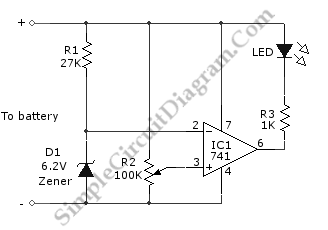# Low Battery Power Indicator

A low-battery indicator circuit is shown in the schematic diagram. This battery indicator circuit will indicate the low battery condition by flashing the LED. This circuit utilize zener diodes as a voltage reference and 741 op amp as voltage comparator to detect if the voltage falls below a preset value. The op-amp will compare the voltage between two inputs, one supplied with a voltage from a high resistance linear potentiometer which act as voltage divider,  and the other is the voltage from zener reference. Here is the schematic diagram of the  circuit:The LED will light to indicate low-battery condition when the op amp’s output becomes low due to the voltage at the  tap of the potentiometer drops below the voltage set by zener. This circuit is designed for 9V battery. If the voltage is lowwer than 9V, the zener must be replaced with smaller voltage zener and current-limiting resistor also replaced with smaller current-limiting resistor. For higher voltage, the larger resistor is used.